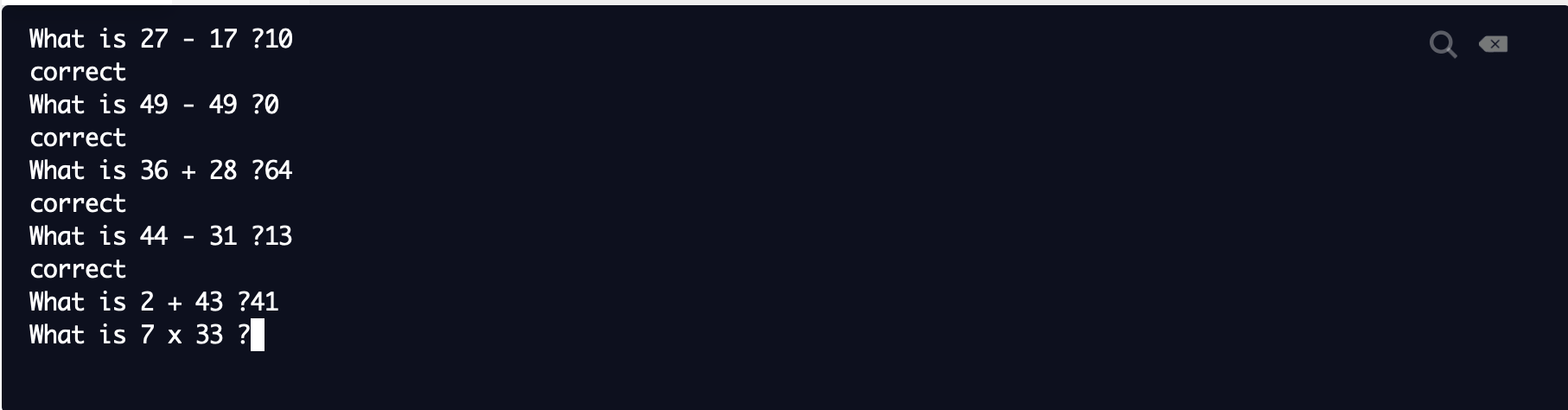← Back to Community
##### Code wont register correct answers
Profile icon
Evan242

Recently I started a small side project where I would try to make a maths quiz using Python 3. The code would generate random equations, each either addition, multiplication, or subtraction. There would also be a score system where you would earn a point for every correct answer. However, each time I run the code it says that the score is zero, even if there are correct answers. Any help in locating the issue would be appreciated

Voters
Profile icon
LyraGriffin
Profile icon
Evan242